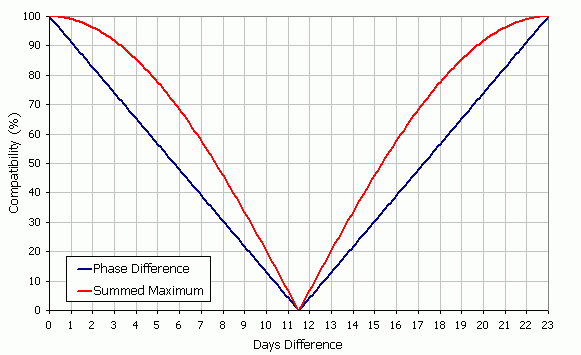Palm Biorhythms Compatibility

Biorhythm Compatibility Calculation

Background

After releasing Palm Biorhythms v1.0, I received many requests to include some form of compatiblity calculation in the next version. As a result I added the compatibility view in v2.0. At the time I hadn't come across a technique to calculate compatibility so I looked at what other programs were doing. It was easy to see that they were using the phase difference between two cycles as a measure of compatibility. Therefore I also adopted this approach.

It wasn't until some months later that I saw that the compatibilty values I was getting didn't agree with a popular web site. This prompted a more detailed look into biorhythm compatilibility and the results were inconclusive.

• There appears to be no definitive way to calculate biorhythm compatiblity. If you know of a definitive way then please contact me.
• Some web pages give formula that appear incorrect.
• One of two approaches are typically adopted.

The upshot is that Palm Biorthythms tries to cover all bases. As of v3.0, it offers two methods to calculate compatilibility. Namely Phase Difference and Summed Maximum. (Please note these are my names for the calculations and I don't make any claims that these are correct, please contact me if you can put me straight.)

The purpose of this page is to describe the details of these calculations and hopefully shed some light on the matter.

Warning: This page assumes the reader has a basic understanding of mathematics and biorhythms. I've tried to keep things as simple as possible and all you need to know is a little trigonometry and that each biorhythm cycle is represented as a sine wave.

Phase difference

Phase difference is the original compatibility method used in Palm Biorhythms. It works as follows, when two person's cycles are in phase their compatibility is 100% when they are out of phase their compatilibility is 0%. Values in between vary uniformly between 100% and 0%.

This definition produces a formula that varies according to the phase difference between two people's biorhythm cycles:

Compatibility % = (200 / p) * Abs(Mod(d, p) - p / 2)

Where:

 d Number of days between the birth dates of the two people being compared p The period of a biorhythm cycle in days. The physical cycle is 23 days, the emotional is 28 days and the intellectual is 33 days. Abs() The absolute value of the number in brackets. The absolute value is the number without its sign. Therefore Abs(-2) is 2. Mod(a, b) The remainder after dividing a by b.

Summed maximum

An alternative method of calculating compatibility involves adding two people's waves together and then finding the maximum value. When the waves are in sync, they combine together to produce a result that's twice as strong; when they are completely out of sync they cancel each other out producing nothing.

I've read on some sites that way to calculate this is to add the two waves together, use differentiation to find the maximum and then divide this result by the value obtained when the waves are in sync. While it is possible to use this approach we can get the same result with much less effort as follows.

We start by adding together two sine waves that are out of sync:

y = sin(x) + sin(x - z)

Here we are assuming that x is a measure of time, z is a measure of the difference between the birth dates of two people and y is the total value (which we want to find the maximum of). We then use the trigonometric identity:

sin(a) + sin(b) = 2 * sin((a + b) / 2) * cos((a - b) / 2)

and substitute a = x and b = x - z into the above. This produces:

y = 2 * cos(z / 2) * sin(x - z / 2)

Recall that we are trying to find the maximum value of y for any value of x. In the above, there's only one term that varies with x, which is sin(x - z / 2). And, in turn, this sine term can vary between -1 and 1 so therefore the maximum value of y is.

ymax = Abs(2 * cos(z / 2))

ymax has a maximum value of 2 which occurs when z = n * 2 * pi (i.e. when the two waves we added together are in sync). This makes the compatibility formula = 100 * ymax / 2.

Finally we express z in terms of the d and p (as defined in the phase difference calculation). If we are using radians to calculate sines and cosines then z = 2 * pi * d / p. Combining everything together gives:

Compatibility % = 100 * Abs(cos(pi * d / p))

Comparison of methods

The graph below shows the how the two calculation methods differ. It plots the compatiblity value for the physical cycle (a period of 23 days) against the phase difference.What's clear is that both methods agree at two points. When the two cycles are in phase the compatibility is 100%, and when the cycles are out of phase the compatibility is 0%. In between the two methods give different results. I don't know which method is correct so Palm Biorthythms can use either method.